### ¡Este curso ha sido revisado!

Para una experiencia de aprendizaje más agradable, le recomendamos que estudie la versión reeditada para móviles de este curso.

- or -

Continue studying this course

• Apuntes
• Revisión por tema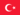BabaJide Martins F. 0 0 Well understood! The accounting equation represents a state of balanced.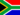Morne V. 0 0 This is another strategy, which is useful when dealing with balance day adjustments. This particular approach emphasises the importance of recognising the dual effect of transactions on the accounting equation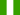Sunday O. 0 0 The accounting equation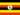Odongo M. 0 0 Accounting -> The accounting equation The accounting equation The accounting equation This is another strategy, which is useful when dealing with balance day adjustments. This particular approach emphasises the importance of recognising the dual effect of transactions on the accounting equation. The accounting equation - The see saw effect The accounting equation represents a state of balance. Initially assets are equal to liabilities plus owner's equity (proprietorship). Once the business commences operations this equation extends to Assets + Expenses = Revenue + Liabilities + Owner's equity (proprietorship). This may be presented in the form of a playground see saw. If the see saw is to be fun it must be kept in balance. There must be equal weight at each end. The left hand side of the seesaw is the debit side and the right hand side is the credit side. Any decrease in the debit side of the seesaw must be matched by a decrease in the credit side, or an increase in the debit side. All changes must be of equal effect.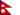Manish K. 0 0 information is clearly given. No question.Penelope M. 0 0 irt's simply a balance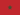Adil N. 0 0 What is the accounting equation?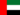Gloria N. 0 0 Any decrease in the debit side of the seesaw must be matched by a decrease in the credit side, or an increase in the debit side. All changes must be of equal effect.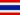Epie E. 0 0 clearly understood.maintaining balance in both sides of the equation.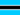Baseli Gloria S. 0 0 oK.